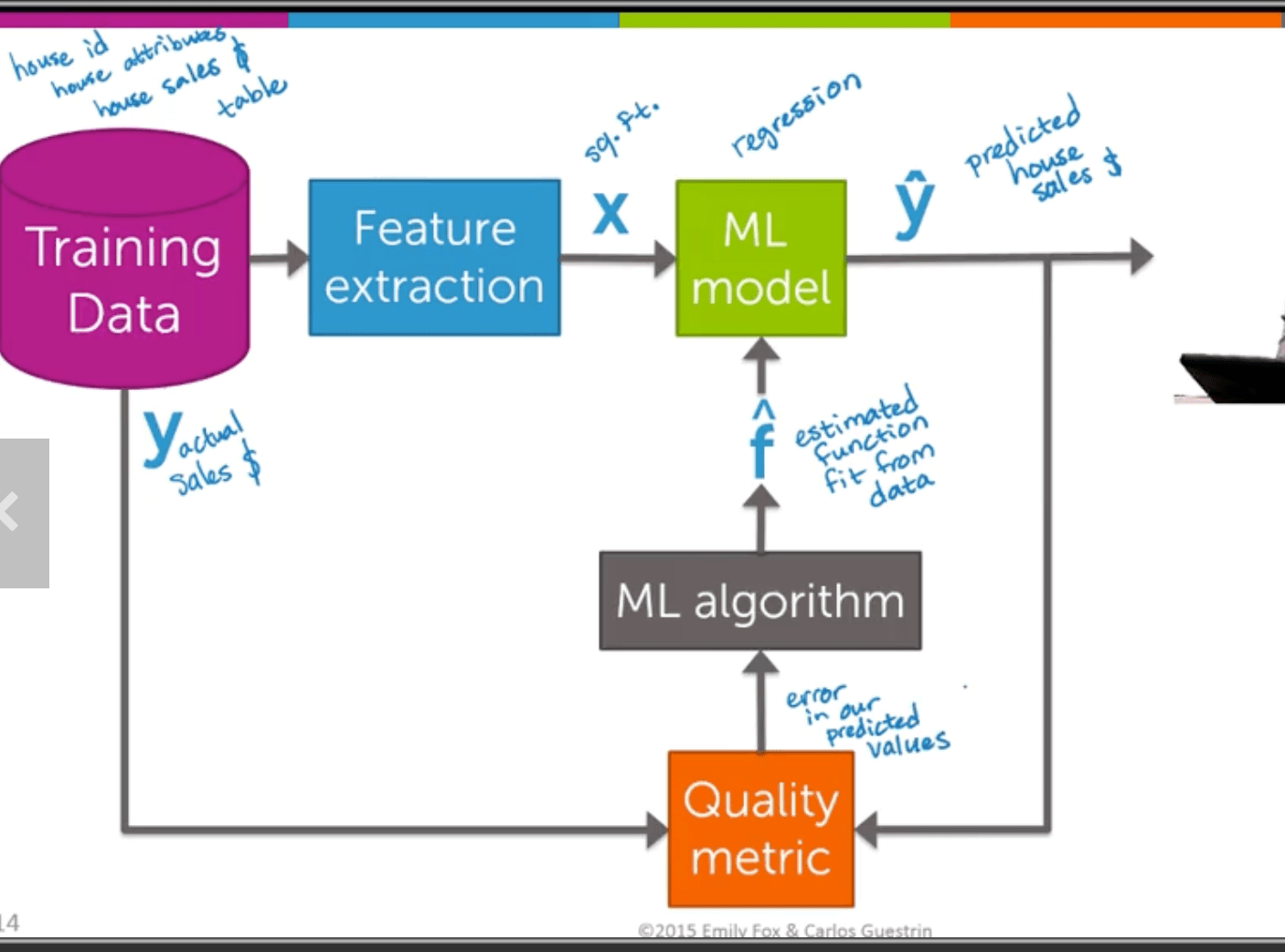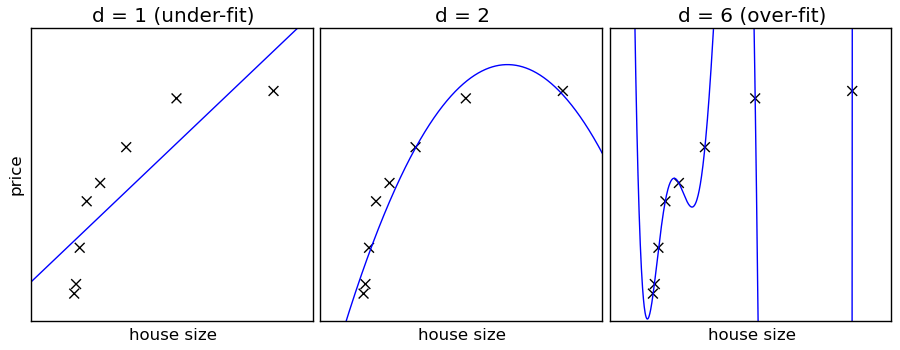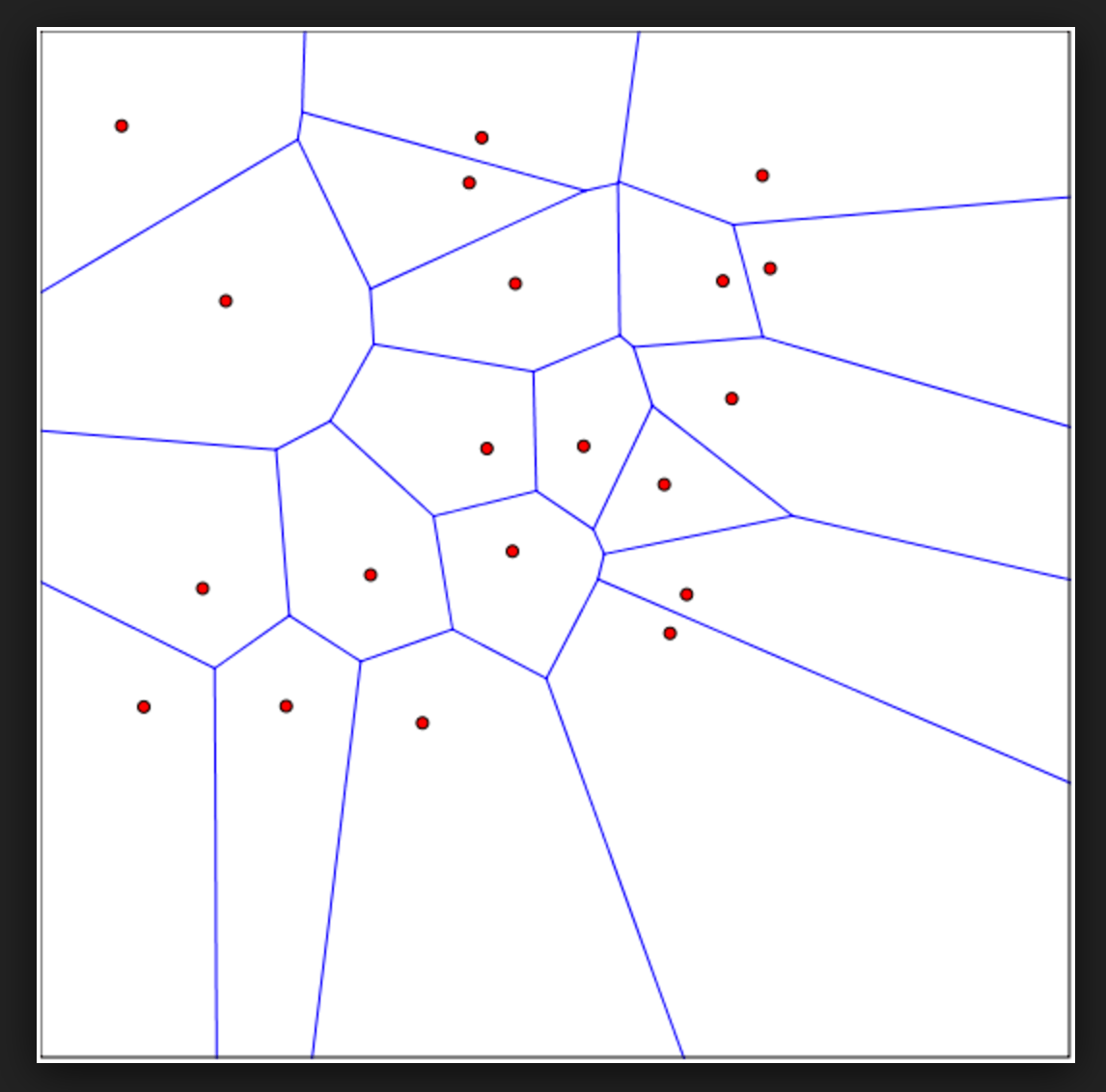# Machine Learning, Part 1: Regression

I’m working my way through a Coursera specialization on Machine Learning. The specialization includes several courses, the first of which provides a high-level overview of ML. I finished that one before I began talking about the coursework on my blog because I didn’t want to identify myself as a student of machine learning until I had actually gone through with something.

Going forward, I’ll share a post on each of the in-depth classes in the specialization. The first in-depth class is called Regression, and it includes six modules. Below I will share a little information about each module, my thoughts on some topics, and links to supplementary reading that I used to deepen my understanding of the concepts in the course.

## Module 1: Linear Regression

The first module covers linear regression, or fitting a line to data. Dr. Fox explained, with this helpful chart, the role that a regression function (or any function that attempts to model a set of data) will play in the cycle of machine learning:We start with a basic concept: our observations of the data are a result of some function, applied to our inputs, plus some amount of error.

observations = function + error = b + mx + error, where b and m are “regression coefficients.”

We measure error with RSS—the residual sum of squares. That is, we find the difference between our model’s estimate and the actual outcome, square it (so that too-low guesses don’t cancel out too-high guesses), and then add up all that wrongness into a sum. We can also use RMSE—root mean square error—to measure the wrongness of our model. Instead of summing the squared errors, we take the square root of all their squares (AKA turn them all positive) and then average them together. The result describes how wrong, in units of the result feature, the model usually is. So if my house price model has an RMSE of \$1200, that means that my model, on average, tends to get house prices wrong by about \$1200 (in either direction). In the most simplistic case (for our purposes, right now, we’ll assume the most simplistic case), our best-fit line for a set of data is the line that incorporates the least wrongness. So how do we find that line?

We can do one of two things:

1. We can used closed-form regression to find the regression coefficients for a line given a set of data. We did this in the homework. I found this resource helpful for doing that.
2. Plot the amount of error (wrongness) that we encounter across all regression coefficients, and then find the lowest one. To find the lowest one, we figure out what direction our error is headed at each point by taking the derivative of the error function. Then we move down that gradient, check it again, rinse and repeat until we reach the point at which the derivative is zero and the second derivative is positive, meaning we have reached a local minimum. This method is called gradient descent, and this article from atomic object helped me understand it. This method is more widely applicable, and often more efficient, than closed-form regression in the real world. More on this later.

## Module 2: Linear Regression with Multiple Features

Before we continue, I will share here the notation that we are using in the course to talk about individual inputs and features. This notation allows us to write things with a common understanding of what they mean:This resource from Dartmouth explained multiple regression in a way that helped me understand what it is, how it works, and why we use the derivative with respect to a given variable to determine the coefficient at that variable. The first three pages cover the concept of multiple regression, and the remaining pages dive into examples from a specific multiple regression problem: examining the effects of many different variables on a person’s wages.

There are two ways to find a solution to a multivariate regression problem: closed-form solution and gradient descent. Closed form solutions do not always exist for these problems, and they can be complicated to calculate. So data scientists frequently use a gradient descent approach instead. The linked resources helped me understand each of these methods for solving multivariate regression problems.

Once I understood the concept of multiple regression analysis and the mechanisms we use to find a multivariate prediction equation, this resource from StatSoft (now owned by Dell) gave me more insight into the challenges associated with applying multivariate regression to real world problems.

## Module 3: Assessing Performance

Next, we talked about the different sources of inaccuracy in a model’s predictions. We discussed irreducible error, bias, and variance. This essay helped me understand the difference between bias and variance and why we might face a tradeoff between them when deciding on the appropriate level of complexity for a model.

The module also included some practice work to select the model complexity with the optimal performance. We split a set of data into training data, validation data, and test data, trained a variety of different models on the training data, ran them against the validation data, and computed the RSS against the validation data. The idea is to select the model with the lowest RSS. Unfortunately, my best solution involved making, training, running, and assessing RSS on every single candidate model. My output looked something like this:

```power 1 predictions yield validation RSS of 6.91195074764e+14
power 2 predictions yield validation RSS of 6.72930696571e+14
power 3 predictions yield validation RSS of 6.10027250649e+14
power 4 predictions yield validation RSS of 6.23430333366e+14
power 5 predictions yield validation RSS of 6.13283570808e+14
power 6 predictions yield validation RSS of 6.53331784575e+14
power 7 predictions yield validation RSS of 6.14978997067e+14
power 8 predictions yield validation RSS of 6.13515765597e+14
power 9 predictions yield validation RSS of 6.23194043494e+14
power 10 predictions yield validation RSS of 6.31328586192e+14
power 11 predictions yield validation RSS of 6.3615241443e+14
power 12 predictions yield validation RSS of 6.37184636893e+14
power 13 predictions yield validation RSS of 6.05078408481e+14
power 14 predictions yield validation RSS of 6.3101294822e+14
power 15 predictions yield validation RSS of 6.26167016623e+14
The most accurate model is the power 13 model, with an rss of 6.05078408481e+14*

* Actual RSS values have been changed to avoid giving away answers to quiz questions.```

I was relieved and disappointed to learn from the above-cited article that we don’t really have a more efficient way to do this. I was relieved because it meant that my solution wasn’t terrible, and I was disappointed because it means that we don’t have something better. I only looked at 15 candidate models, and the program took several seconds to run. What if we wanted to test a thousand different candidate models? That could take a lot of time.

Also, the best solution for a data science question might not be just one of a set of candidate models, but rather a blend of a few different ones. The earlier modules of this course mentioned just such a case, wherein a team called BellKor Pragmatic Chaos won the Netflix Prize for a ratings prediction algorithm that outperformed Netflix’s own CineMatch by about 10%. Their algorithm blended over a hundred component algorithms together.

## Module 4: Ridge Regression

Although bias and variance were the topics of the previous module, it was this module that cemented my understanding of them.

Ridge regression gives us a way to assess whether a model is overfit or not: that is, whether our model performs better on the training data but worse on the test data than a less complex model. Such a performance indicates a large amount of variance—that is, our measure of how much our model depends on the specific subset of data used to train it, as opposed to the overall trends that we might see in other, similar (but not exactly the same) data. Overfit models tend toe exhibit low bias but high variance, while less complex models might have less variance, but introduce more bias.

At any rate, something really interesting happens when a linear regression model gets overfit: things get really steep.*Thank you to the scikit-learn tutorial for this image.

The overfit model on the right has way steeper slopes than the others. It’s those really steep slopes all over the place, manifested as extremely large coefficients on each of our features, that warn us of potential overfit.

As it happens, we can introduce new parameters in the training of our models to bias the training against these large coefficients and choose simpler models that better generalize to other datasets. One such parameter is called the L2 Penalty, and it penalizes the model based on the sum of the squared values of the coefficients. This module was about that. The other parameter for this, the L1 Penalty, penalizes the model based on the sum of the absolute values of the coefficients. The next module will cover this one.

I confess I don’t understand yet why one would want to use both the L2 Penalty and the L1 Penalty. I can see from the math that the L2 penalty’s handicap on the model would exponentially increase as the coefficients linearly increased, while the L1 Penalty’s handicap would increase linearly along with the coefficients. But I’m missing the ‘so, what?’ here. I suppose I’ll understand this better next week.

## Module 5: Feature Selection & Lasso

As it turns out, the L1 Penalty comes in very handy for feature selection. Suppose we have a set of data where each data point has lots and lots of features that all might contribute to the outcome. How do we decide which of these are important? It’s computationally expensive to run models with tons and tons of features, and it’s even more computationally expensive to try to choose the best model from every single possible combination of features. Also, it’s hard to wrap our heads around models that use so many features. So we look for efficient ways to select the features that most reliably influence our outcomes, and leave out the others.

There are a lot of techniques to do this. The one described in this module, LASSO, involves learning the coefficients for each feature in the model on training data and knocking out the smaller ones, so only the heaviest predictors of the outcome remain. We choose an L1 penalty and then calculate a wrongness amount (referred to as rho, usually) on each of our weights. The wrongness amount considers both the predictive error and the size of the coefficient. If rho falls between the negative and positive halves of the L1 penalty, then we make that coefficient zero and knock that feature out of the model. If rho is further from zero than half the magnitude of the L1 penalty, we move it towards zero by a factor of half of the L1 Penalty. We iteratively perform coordinate descent on each weight until all of the weights stop changing by a factor of more than some tolerance amount that we choose.

The result is a model with fewer features, and a higher L1 Penalty means a less fully-featured model. These simpler models also tend to have higher RSS, so we face the familiar tradeoff between consistent wrongness (bias) and variable wrongness (variance). I’m guessing this tradeoff is what data scientists get paid the big bucks to face.

## Module 6: Nearest Neighbors and Kernel Regression

This final module of the course on regression covered our first non-parametric method for regression modeling: K nearest neighbors. We begin with a set of training data, and we make predictions about new observations based on which of the training data points the new points are closest to. This illustration of a training dataset outlines the regions closest to each point. So in a 1-nearest-neighbors model, we would predict stuff about a new data point in one of these regions by looking at the values for the training data point of that region.*Image courtesy of the Machine Learning course at my alma mater, apparently.

This might seem like a primitive approach to making predictions, but it’s actually pretty close to how our brain predicts things. When we want to understand an unknown situation, we look for a precedent. That’s what this is.

We can also use this approach in a more sophisticated fashion. For example, we can predict values on a new observation by averaging the values of its 5 nearest neighbors, or 10 nearest neighbors, or 100 nearest neighbors. Ot we can even use all the training data to make predictions on every new point, and weight their influence according to which points are closest to the new point. That’s the essence of kernel regression: take some subset of the points in the training data, and weight that subset differently according to its distance to the query point. There are several different strategies for this—this Wikipedia article shows ten different possible kernels we could use.  As it turns out, which one you choose matters less than the lambda you choose (how wide/fat you want to make your kernel). Larger lambdas help smooth over the jumps in the value predictions that happen when some data points jump into the prediction scope and others jump out. A lambda that is too wide, though, can oversmooth the predictions.

Data analysts choose values for k and lambda, and choose a kernel, by training many different k-nearest-neighbors models on a training data set and then measuring their relative accuracy against a validation data set. Then the most accurate one might be used to predict on a test data set. This technique echoes throughout regression models and throughout modeling in general. We are likely to see more of it in the upcoming courses :).

This site uses Akismet to reduce spam. Learn how your comment data is processed.